Mathematica Functions and Basic Operations on Expressions

Mathematica Functions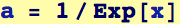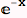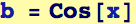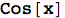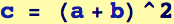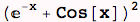Alternative Syntax for Functions (There are many ways to do the same thing)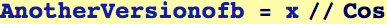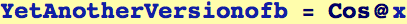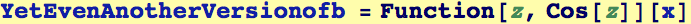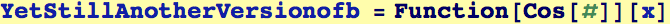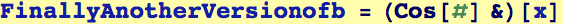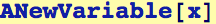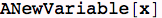Mathematica Operations on expressions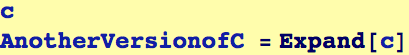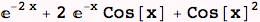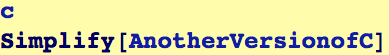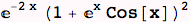Calculus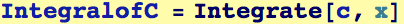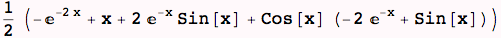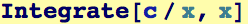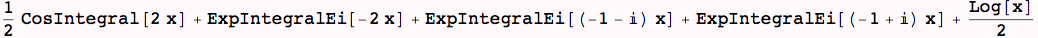Getting information (part 1)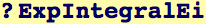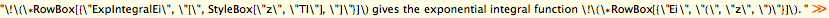Created by Wolfram Mathematica 6.0  (28 August 2007)